# How to Calculate Average If Criteria Not Blank/Ignore Blank Cell

In daily work we often need to calculate the average of some numbers based on given conditions or criteria. To calculate average with criteria, we can apply AVERAGEIF of AVERAGEIFS function. AVERAGEIFS function can handle more than one group of criteria range and criteria. In this article, we will show you how to calculate average of numbers whose corresponding cells in another group are not blank. Thus, we need to know some knowledge of AVERAGEIFS function.

In this article, we will let you know the syntax, arguments, and basic usage about AVERAGEIFS function, and apply it to build a formula, also let you know the calculation steps of the formula.

## EXAMPLE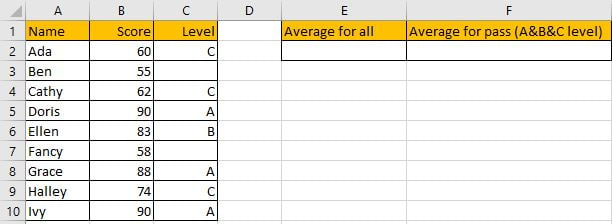Refer to “Score” column, some numbers are listed in range B2:B10. “Level” column is used for providing a level based on scores. In E2 and F2, we calculate the average of all scores and qualified scores separately. To calculate average of all scores, we can directly use AVERAGE function. To calculate average of qualified scores (cell is not blank in Level group), we need to add criteria to filter them, we can apply AVERAGEIFS function, it is also one of the most common used functions in Excel.

First, In C2, enter “=AVERAGE(Score)”. Then press Enter, AVERAGE function returns 73.33333333.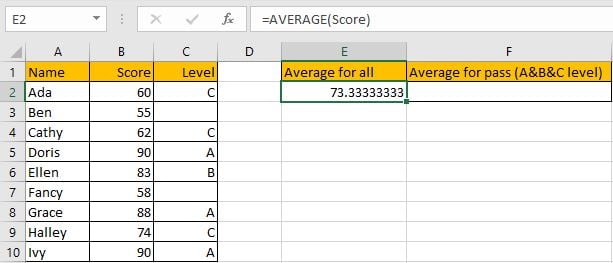You can adjust decimal places by “Increase Decimal” or “Decrease Decimal” in “Number” section.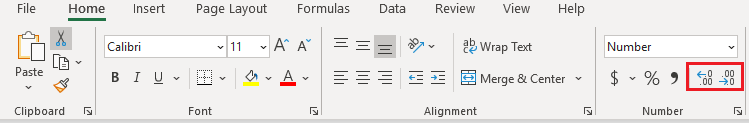Keep clicking on “Decrease Decimal” button till decimal places is ok for you. Keep two decimal places in this case.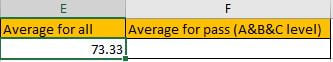Now we can apply AVERGAEIFS function to calculate the average of qualified scores.

Before creating the formula, name range “B2:B10” to “Score”, “C2:C10” to “Level” in Name Box.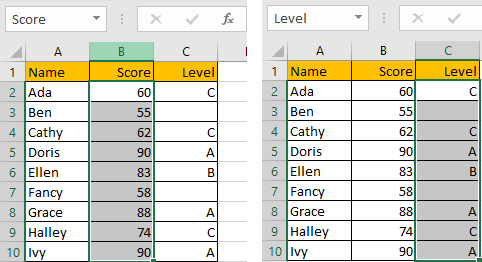## CREATE A FORMULA with AVERAGEIF FUNCTION

### 1. STEPS

Step 1: In F2, enter the formula =AVERAGEIFS(Score,Level,”<>”).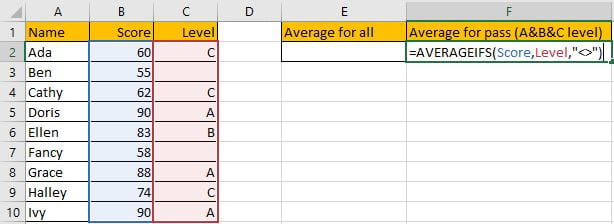Step 2: Press Enter after typing the formula.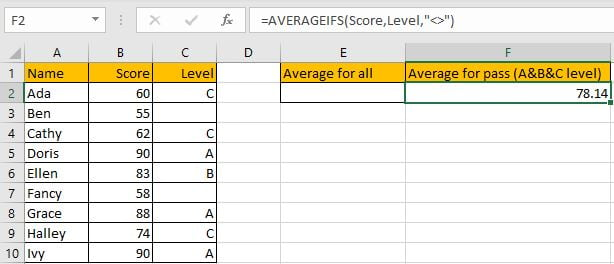Only B2, B4, B5, B6, B8, B9 and B10 meet our given criteria “level is A or B or C”, so we calculate the average of numbers in above cells, total 7 numbers. (60+62+90+83+88+74+90)/7=78.14285714, keep two decimal places 78.14. The formula works correctly.

### 2.FUNCTION INTRODUCTION

AVERAGEIFS function is AVERAGE+IFS. It returns the average of some numbers in a range based on one or more given conditions or criteria.

Syntax:

`=AVERAGEIFS(average_range, criteria_range1, criteria1, [criteria_range2], [criteria2],…)`

It supports wildcards like asterisk ‘*’ and question mark ‘?’, also supports logical expressions like ‘>=’,’<=’. If wildcards or logical operators are required to build criteria, they should be enclosed into double quotes (““). In this case we entered “<>” to represent criteria.

### 3.ALL ARGUMENTS

AVERAGEIFS – Average Range

This example is very simple, we have only one average range A2:A10.

In the formula bar, select named range “Score”, press F9, values in this range are expanded in an array.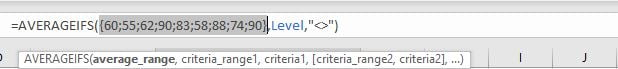AVERAGEIF – Criteria Range

The criteria range is C2:C10.

In the formula bar, select named range “Level”, press F9, values in this range are expanded in an array.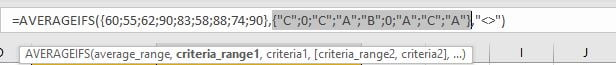AVERAGEIF – Criteria

The criteria in our case is “<>”, in this case it represents cells are not blank. As logical expression is used, so we add “” to quote “<>”.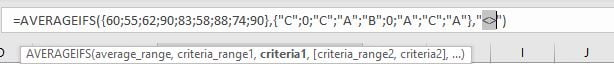### 4.EXPLANATION

After expanding values, the formula is displayed as:

`=AVERAGEIFS({60;55;62;90;83;58;88;74;90},{"C";0;"C";"A";"B";0;"A";"C";"A"},"<>")`

Note: 0 is recorded in this array to represent blank cells.

In the formula, the criteria is “<>” (“not equals to” operator), based on this criteria, cells without any level in “Level” column cannot meet our condition, so for the corresponding values in the average range, they are excluded in calculation.

{60;55;62;90;83;58;88;74;90} -> {60;0;62;90;83;0;88;74;90} – Ignore blank cells

Now this new array only contains numbers. We can calculate the average now (60+62+90+83+88+74+90)/7=78.14.

## SUMMARY

1. AVERAGE function is used for returning the average of some numbers in Excel.

2. AVERAGEIFS function returns the average of some numbers refer to one or more given condition or criteria.

3. AVERAGEIFS can support wildcards, logical expressions.

### Related Functions

• Excel AVERAGE function
The Excel AVERAGE function returns the average of the numbers that you provided.The syntax of the AVERAGE function is as below:=AVERAGE (number1,[number2],…)….
• Excel AVERAGEIF function
The Excel AVERAGEAIF function returns the average of all numbers in a range of cells that meet a given criteria.The syntax of the AVERAGEIF function is as below:= AVERAGEIF (range, criteria, [average_range])….
• Excel AVERAGEIFS function
The Excel AVERAGEAIFS function returns the average of all numbers in a range of cells that meet multiple criteria.The syntax of the AVERAGEIFS function is as below:= AVERAGEIFS (average_range, criteria_range1, criteria1, [criteria_range2, criteria2],…)….

Related Posts

Break ties with helper COUNTIF and column

Suppose you got a task to adjust the values that contain the ties; what would be your first attempt to break the ties of the given value? If you are wondering about doing this task manually, let me add that ...

Calculate Total Cost with Excel VLOOKUP Function

In today's article we will show you how to calculate the total cost for a given weight using the Excel VLOOKUP function. This function will help us to find the appropriate unit price for that weight and then we can ...

Excel Formulas To Calculate The Bond Valuation

Assume that you've been assigned a task of calculating bond valuation; so if you are new to Ms Excel or do not have much experience with it, then I am pretty sure about it that doing this task manually might ...

Calculate Cumulative Totals with Excel SUM Function

Today, through a simple example, we will show you how to use one of the most common-used Mathematical functions in excel, the SUM function, to add up the sum. In our daily life, we keep an account of what we ...

BMI Calculation Formula In Ms Excel

You might have come across a task in which you were assigned to make BMI calculations of the supplied numbers, and you may be looking for an efficient approach to accomplish this process rather than doing BMI calculations manually, by ...

Calculate The Period of Loan or Investment in Excel

In our daily life, most of us will invest or take a loan, so we need to master some simple financial functions to calculate the period needed for loans or investments. If you work as an accountant, you need to ...

Working Time Calculation Based on Timesheets

In the office, a special machine record the time when you start working (clock in office) and when you finish working (clock out of office). We can calculate the total working time by subtracting the end working time from the ...

Sort/Rank Numeric Values with Duplicate Values Exist

Excel built-in RANK function can sort a set of values. If there are duplicate numbers, then the rank number is also duplicated. See the following example: There are two numbers “100” in range A2:A9, they are both the third largest ...

Calculate Days Open in Excel

If you want to know how to Calculate days in Excel, there are some formulas that you can use to do so. For example, you can use the DAYS function in Excel to find the number of days between two ...

Calculate Grades With VLookup in Excel

Why Should You Calculate Grades With VLookup Excel? If you're looking for a simple way to find out a student's grades, VLOOKUP Excel can do the trick. This function uses a lookup table to find the values and sort them ...

Sidebar